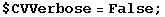7.3. ComponentValue and TensorValues with independent values

Until now, the second list of the FoldedRules contained only trivial replacement rules such as. It is very simple to specify values for the LHS, we just have to call ComponentValue with two arguments:

In:=Out=In:=Out=Notice what happens if we specify a dependent component

In:=Out=First the dependent rule is added and then the value (with a possible sign) is assigned to the corresponding independent component:

In:=Out=ComponentValue does not allow the user to introduce an inconsistent value

In:=Out=We switch the rule generation messages off

In:=ComponentValue is threaded on pairs of lists when it has two arguments. This allows us to generate all independent rules with just one command.

In:=Out=In:=Out=In:=Out=Created by Mathematica  (May 16, 2008)